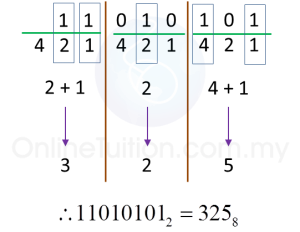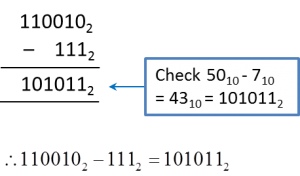# 1.2 Number Bases, SPM Paper 1 (Short Questions)

Question 8:
Express 110101012 as a number in base eight.

Solution:Question 9:
Express 100001102 as a number in base eight.

Solution:Question 10:

Solution:Question 11:
Express each of the following as a number in base two.
(a) 26 + 24 + 1
(b) 25 + 23 + 2 + 20

Solution:
$\begin{array}{l}\text{(a)}\\ {2}^{6}+{2}^{4}+1\\ =\underset{_}{1}×{2}^{6}+\underset{_}{0}×{2}^{5}+\underset{_}{1}×{2}^{4}+\underset{_}{0}×{2}^{3}+\underset{_}{0}×{2}^{2}+\underset{_}{0}×{2}^{1}+\underset{_}{1}×{2}^{0}\\ ={1010001}_{2}\end{array}$

$\begin{array}{l}\text{(b)}\\ {2}^{5}+{2}^{3}+2+{2}^{0}\\ =\underset{_}{1}×{2}^{5}+\underset{_}{0}×{2}^{4}+\underset{_}{1}×{2}^{3}+\underset{_}{0}×{2}^{2}+\underset{_}{1}×{2}^{1}+\underset{_}{1}×{2}^{0}\\ ={101011}_{2}\end{array}$

Question 12:
State the value of the digit 2 in the number 324175 , in base ten.

Solution:
 3 2 4 1 7 Place Value 54 53 52 51 50
Value of the digit 2
= 2 × 53
= 250

Question 13:
101102 + 1112 =

Solution:Alternatively, use a scientific calculator to get the answer directly.

Question 14:
1100102 – 1112 =

Solution:Alternatively, use a scientific calculator to get the answer directly.